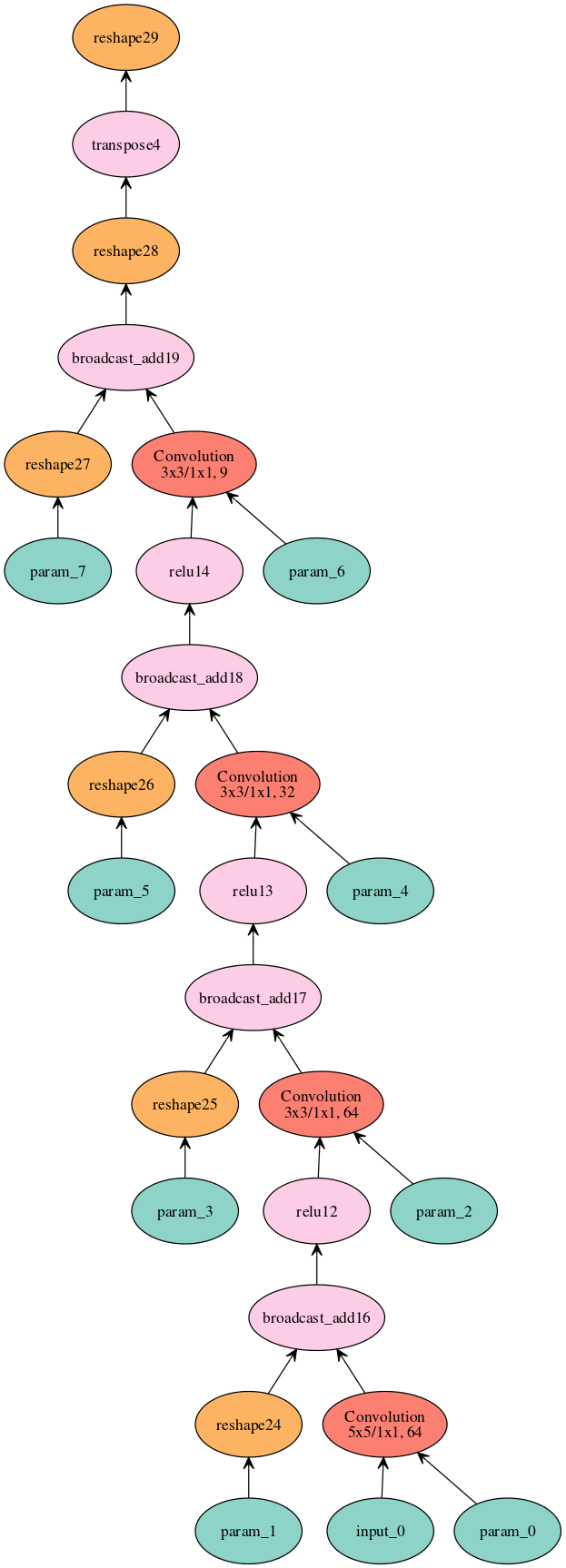Importing an ONNX model into MXNet¶

In this tutorial we will:

• learn how to load a pre-trained ONNX model file into MXNet.

• run inference in MXNet.

Prerequisites¶

This example assumes that the following python packages are installed: - mxnet - onnx (follow the install guide) - Pillow - A Python Image Processing package and is required for input pre-processing. It can be installed with pip install Pillow. - matplotlib

from PIL import Image
import numpy as np
import mxnet as mx
import mxnet.contrib.onnx as onnx_mxnet
from matplotlib.pyplot import imshow

Fetching the required files¶

img_url = 'https://s3.amazonaws.com/onnx-mxnet/examples/super_res_input.jpg'
model_url = 'https://s3.amazonaws.com/onnx-mxnet/examples/super_resolution.onnx'

To completely describe a pre-trained model in MXNet, we need two elements: a symbolic graph, containing the model’s network definition, and a binary file containing the model weights. You can import the ONNX model and get the symbol and parameters objects using import_model API. The paameter object is split into argument parameters and auxilliary parameters.

sym, arg, aux = onnx_mxnet.import_model(onnx_model_file)

We can now visualize the imported model (graphviz needs to be installed)

mx.viz.plot_network(sym, node_attrs={"shape":"oval","fixedsize":"false"})Input Pre-processing¶

We will transform the previously downloaded input image into an input tensor.

img = Image.open('super_res_input.jpg').resize((224, 224))
img_ycbcr = img.convert("YCbCr")
img_y, img_cb, img_cr = img_ycbcr.split()
test_image = np.array(img_y)[np.newaxis, np.newaxis, :, :]

Run Inference using MXNet’s Module API¶

We will use MXNet’s Module API to run the inference. For this we will need to create the module, bind it to the input data and assign the loaded weights from the two parameter objects - argument parameters and auxilliary parameters.

To obtain the input data names we run the following line, which picks all the inputs of the symbol graph excluding the argument and auxiliary parameters:

data_names = [graph_input for graph_input in sym.list_inputs()
if graph_input not in arg and graph_input not in aux]
print(data_names)
`python
mod = mx.mod.Module(symbol=sym, data_names=data_names, context=mx.cpu(), label_names=None)
mod.bind(for_training=False, data_shapes=[(data_names,test_image.shape)], label_shapes=None)
mod.set_params(arg_params=arg, aux_params=aux, allow_missing=True, allow_extra=True)

Module API’s forward method requires batch of data as input. We will prepare the data in that format and feed it to the forward method.

from collections import namedtuple
Batch = namedtuple('Batch', ['data'])

# forward on the provided data batch
mod.forward(Batch([mx.nd.array(test_image)]))

To get the output of previous forward computation, you use module.get_outputs() method. It returns an ndarray that we convert to a numpy array and then to Pillow’s image format

output = mod.get_outputs()
img_out_y = Image.fromarray(np.uint8((output.asnumpy().clip(0, 255)), mode='L'))
result_img = Image.merge(
"YCbCr", [
img_out_y,
img_cb.resize(img_out_y.size, Image.BICUBIC),
img_cr.resize(img_out_y.size, Image.BICUBIC)
]).convert("RGB")
result_img.save("super_res_output.jpg")

You can now compare the input image and the resulting output image. As you will notice, the model was able to increase the spatial resolution from 256x256 to 672x672.

Input Image | Output Image |
———– | ———— |||